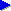# for loops

In the previous section of this Java tutorial, we intoduced the very important notion of variables. Now, with the help of variables, we're going to write a short program that uses another very important construct called a loop.

A loop is essentially a section of program that repeats over and over for some given number of times, or while some given condition still holds. Specifically, we're going to look at what is generally called a for loop. This is generally a loop that repeats for all the values of a particular variable between a given range.

For example, let's say we want to write a simple program that prints the 7 times table. As is customary, we'll consider displaying "7 times n", for all values of n between 1 and 12. So what we write looks something like this:

```for (int n = 1; n <= 12; n++) {
int result = 7 * n;
System.out.println("7 times " + n + " = " + result);
}
```

Hopefully the second and third lines are starting to become familiar now. These define what we do for a particular value of n: each time, we declare a variable called result, and we set it to have the value of seven times the given value of n. Then on the next line, we print out the result, along with some text. We still haven't really explained in detail this use of System.out.println(), but this and other examples we've seen are hopefully starting to give you a rough idea of how it works.

Around these two lines, we have a "wrapper" that turns it into a for loop. On the first line is the actual for statement. If you look carefully, you can see that it has the following format:

for ( declaration ; condition ; update action )

We can describe the three parts in more detail as follows (note that strictly speaking, all of these parts are optional, but usually, they're all included, as here):

• the declaration is an initial variable declaration that is made at the very start of the loop;
• the condition is the condition that must be met for us to continue repeating the code each time (this condition is actually checked before each repetition);
• then, the update action specifies what we do at the end of each repetition of the loop.

In this case, we want to consider all the values between 1 and 12 inclusive. In order to do so, we need to keep repeating the loop as long as n is less than or equal to 12. Notice the use of <= which means this. Then, we want to add 1 to the value of n ready for the next repetition. Recall from the section on using variables that a shorthand for adding 1 (or incrementing) is to use ++. But we could also have written n = n + 1 if we'd wanted to.

The curly braces (the symbols { }) define a block. A block, sometimes called a scope block, defines a "unit of program". In this case, the block directly after the for statement defines the section of code that is to be looped. If we didn't put the braces, then Java wouldn't know which precise set of lines we wanted to repeat. (In fact, it would then assume that it was just the single line directly following the for statement that we wanted to repeat; but in general, it's good practice to put the braces, however many lines are inside them.)

To understand how the loop works, it may be worth writing a list of the actions in the order they occur:

• first, a new variable n is set up, and set to an initial value of 1;
• then, we consider the first repetition of the loop: we check that the condition is met (n <= 12), decide that it is, and start executing the loop code (the part inside the curly braces);
• after executing this code, we perform the update action— in this case, we increment n so that its value is now 2;
• then, we consider the loop condition again; it is still less then or equal to 12, so we go through the loop again;
• this cycle repeats a few times...
• ...and eventually, we'll go through the loop with n having the value 12; after running the loop with this value, we still update n as usual, so that n is now 13;
• now, we consider running through the loop again, but this time, the condition n <= 12 is not met, so we stop executing the loop. The program will continue executing at whatever line is directly after the loop (i.e. directly after the curly brace at the end of this example).

## Running the times table program

Once you've understood the explanation of the looping program above, you probably want to try running it. To run the program, you need to type it into the IDE (editor) you're using to work with Java. In our guide to getting started with Java and Netbeans, we showed how to add your first line of Java code and then run the program. You need to follow that guide, putting all four lines of code in the place indicated by the red arrow, where you previously just had a line to output Hello World!.

## Next...

On the next pages, we look at:nested for loops: a technique of using more than one for loop for cycling over combinations of things;if statements, which allow us to introduce conditions or decisions into our program.

If you enjoy this Java programming article, please share with friends and colleagues. Follow the author on Twitter for the latest news and rants.